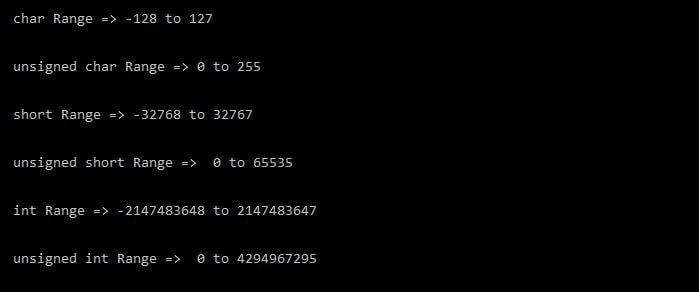# C Program to find the Range of Fundamental Data TypesWhen you are working on data types then you should know the range of the data types. If you are not clear about the range then you might get the undefined behavior.

There is also two header file in c (limits.h and float.h) to provide the range but you can also create an own function to get the range of the data type.

But it is my advice don’t use your own function to get the range of the data types. I am writing this article because some student asks me to write a program to find the range of fundamental data types.

### Steps to find the range of the data types

1. Calculate the size of the data type in bytes using the sizeof operator.
2. Convert the bytes into bits.
2. For signed data types, use formula -2^(n-1) to (2^(n-1))-1.
3. For unsigned data types, the range will be 0 to (2^n) – 1.

Where n is the number of bits of the data type.

#### See the below example program,